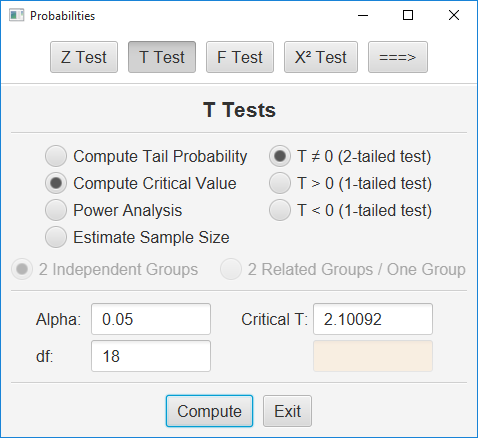# Statistical Calculator

### Stats Tables

One of the utility programs packaged in Stats Homework is a statistical calculator called Stats Tables.  This program will largely replace all the statistical tables found at the back of your textbook:In this example, you are working with a T test with 18 df, you are conducting a two-tailed test, and your alpha level is .05.  You would compare your computed T value to 2.101.

This program can give you the p value (i.e., significance level) of a test statistic and the critical value of that statistic.  With most statistics, it can also conduct a power analysis or give you the required sample size for a given level of power.  It covers all the basic distributions that are considered in a basic statistics course.

Return to Home Page